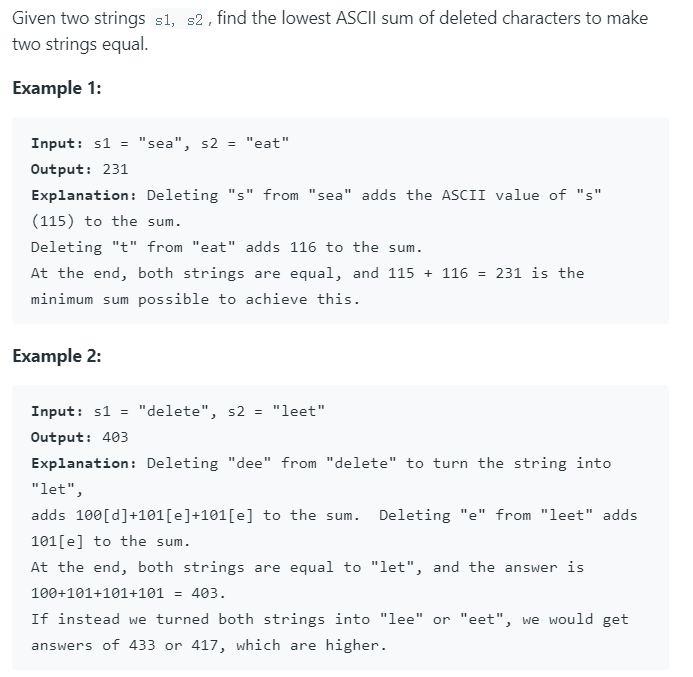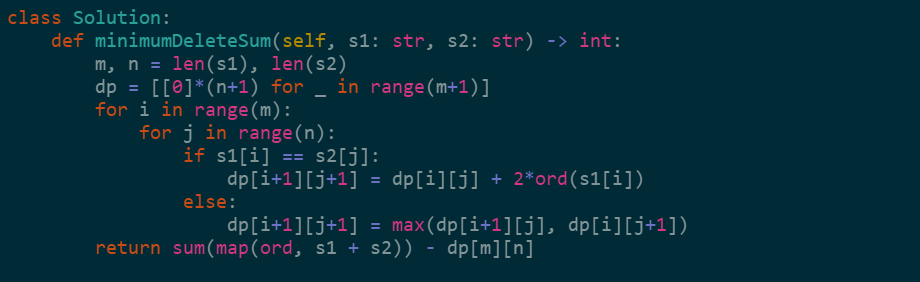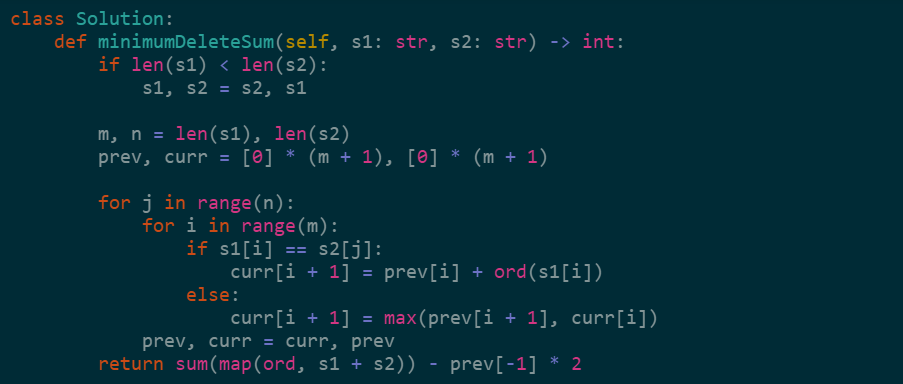# leetcode 712 Minimum ASCII Delete Sum for Two Strings 详细解答

• 时间:

## leetcode 712 Minimum ASCII Delete Sum for Two Strings 详细解答F( i+1, j+1 ) 表示字符串 s1[0 … i] 和 s2[0 … j]相同子序列ASCII的和。

$F( i+1, j+1 )=\begin{cases} F( i, j ) + 2*ASCII(s1[i])& s1[i]=s2[j] \\ max(F(i,j+1), F(i+1,j)) & s1 [i] != s2[j] \\ \end{cases}$